Next: Pulse Pattern Optimization Up: ACF Determination Previous: ACF Determination

### Double-Pulse

The autocovariance function is the basis for the determination of the Doppler velocity power spectrum of the scattering region or equivalently, assuming that only a single velocity component is present, the determination of the mean Doppler velocity of the scatterers.

To determine the complex ACF values at a specific lag-time, two pulses that are separated by a time interval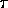are considered. Since the analysis that follows deals with proportional values only, constants have been dropped. As shown in Section 3.1, voltages at the receiver depend on the Fourier component of the density fluctuation with wave vector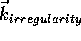(or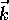in short). Therefore the voltages of the two measurements are (see Figure 3.4):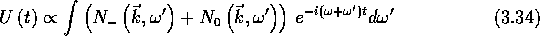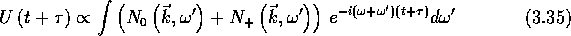where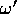is a variable of integration and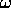is the frequency of the radar wave.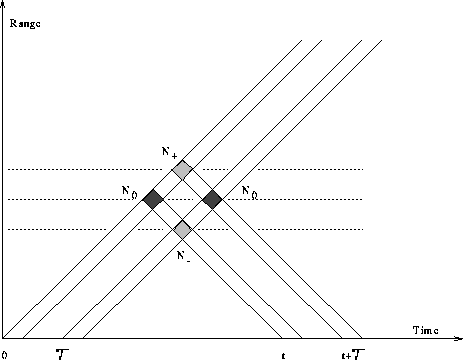Figure 3.4: Range-time diagram for two pulses seperated by a timeThe backscattered signal from two pulses can contain the three components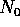,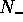and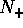as indicated in Figure 3.4.is the electron density spectrum illuminated by the first pulse and measured at time t or illuminated by the second pulse and measured at time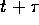is the electron density spectrum illuminated by the second pulse and measured at time tis the electron density spectrum illuminated by the first pulse and measured at timeWe attempt to gain information about the spectrum.

The received voltages can be mixed with two phase-coherent transmitted frequency components that differ by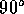in their phase. As an example the voltage measurements at time t are described in Equation 3.36 and 3.37. The voltages at timeare derived the same way. These voltages are taken to be different and correspond to the real and imaginary component of the signal.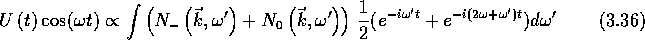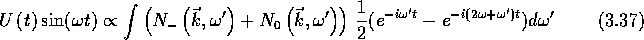Low-pass filtering can then be used to remove the HF part corresponding to a frequency of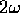. We are left with the two voltages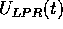and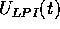, one corresponding to the real and one to the imaginary component of the complex voltage measurement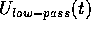:The voltages are averaged over several measurements. We denote the averaging process by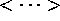. Furthermore a ratio between two voltages differing by the lag time, that isand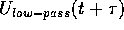, is formed. Using the following short-hand notation for the electron density spectra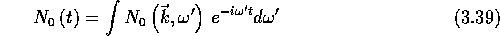the ratio of the two time separated and averaged voltage measurements can be expanded and written as follows: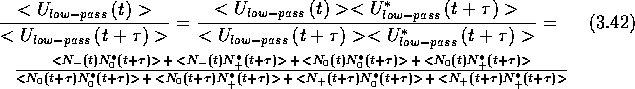where * denotes a complex conjugate value. The information about the Doppler shift is contained in the term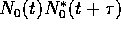. It is not possible to determine this term by a single measurement since all terms in the above ratio are of the same order. But since we are dealing with averaged values uncorrelated values will go to zero in the mean. Thus, we assume that the mean values of the received voltages are zero, and the densities,andare independent. The term defining the ACF of the power spectrum belonging toat lag, that is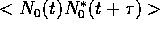, is then separable easily from the ratio [And80, p. 11-15,]. To determine a complete ACF as a function of, this procedure has to be repeated for different.Next: Pulse Pattern Optimization Up: ACF Determination Previous: ACF Determination

Andreas Schiffler
Wed Oct 9 10:05:17 CST 1996